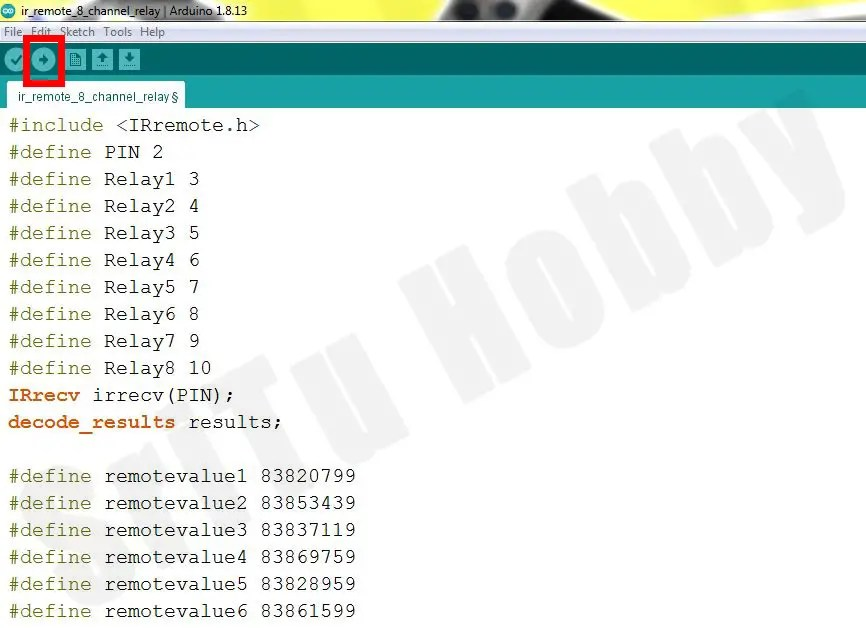# How to use IR Receiver and IR Remote | IR RECEIVER module

##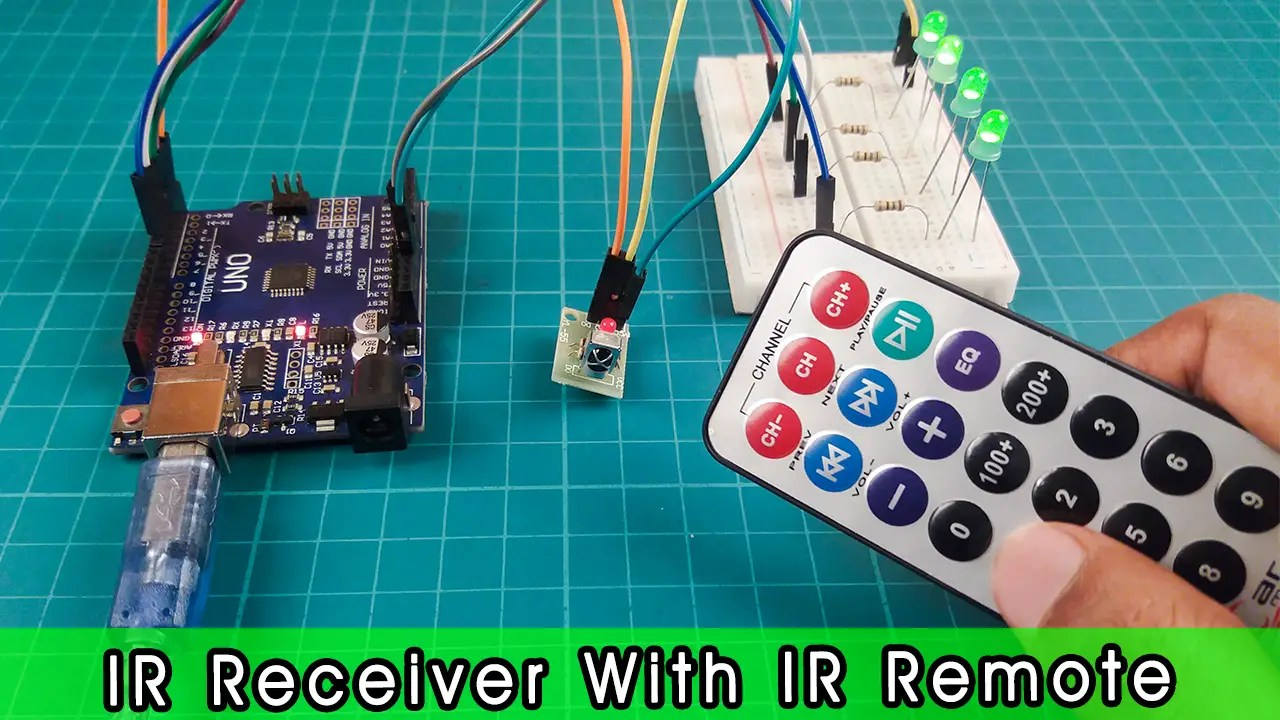Hello guys, welcome back to my blog, This tutorial includes how to use IR receiver and IR remote with Arduino. This tutorial also covers how to turn an LED bulb and buzzer on and off with an IR remote using an IR receiver. Finally, there are step-by-step instructions on how to enable and disable several LEDs using an IR module (IR Receiver and IR Remote) and how to turn ON and OFF an 8-channel relay module.

What is IR Receiver and IR Remote?

The IR receiver works by using IR rays. These IR rays are also known as Infrared rays. These are invisible to our naked eye. Also, these rays have a longer wavelength than visible light. Therefore, these infrared rays are more suitable for wireless communication. We can see this technology in various appliances in the house such as TV, radio, fan. They remotely emit IR rays and are captured by the IR receiver in the electrical device. So we can do the relevant work. This IR receiver operates in the 38kHz band. For this reason, IR rays can be detected via the IR remote, bypassing other IR rays in the environment. This IR receiver also includes a filter called band-pass. It only emits 38KHz IR rays. The frequency is then converted to a binary number.

The different types of IR receivers are listed below.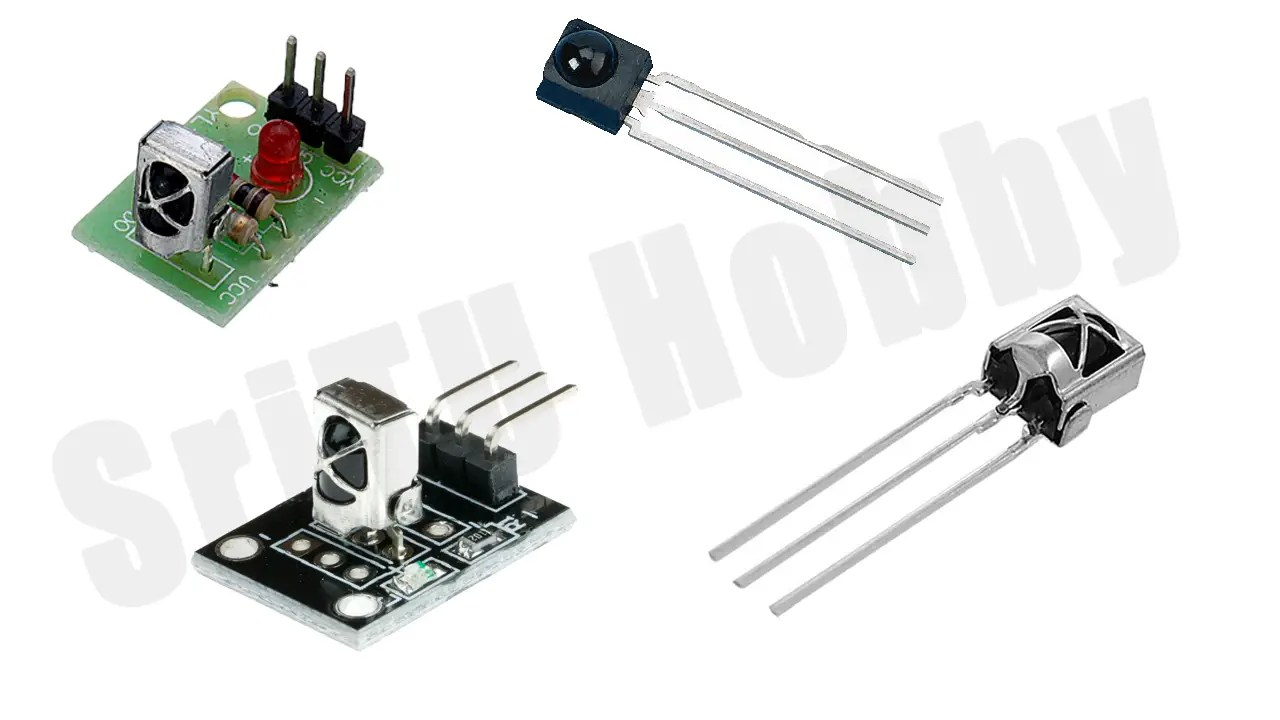We can see three main pins in these.Signal –     Get IR remote signals

VCC –       This should provide a 2.5v – 5V potential.

GND –      Cathode terminal should be connected to this.

Well, now we will learn how this IR receiver works with Arduino. The necessary accessories are given below. Here we show you how to ON/OFF an LED and Buzzer using any IR remote in the house using an IR receiver.

OK, let’s do this project step by step.

### Step1

Let us first identify these devices.
Arduino UNO board.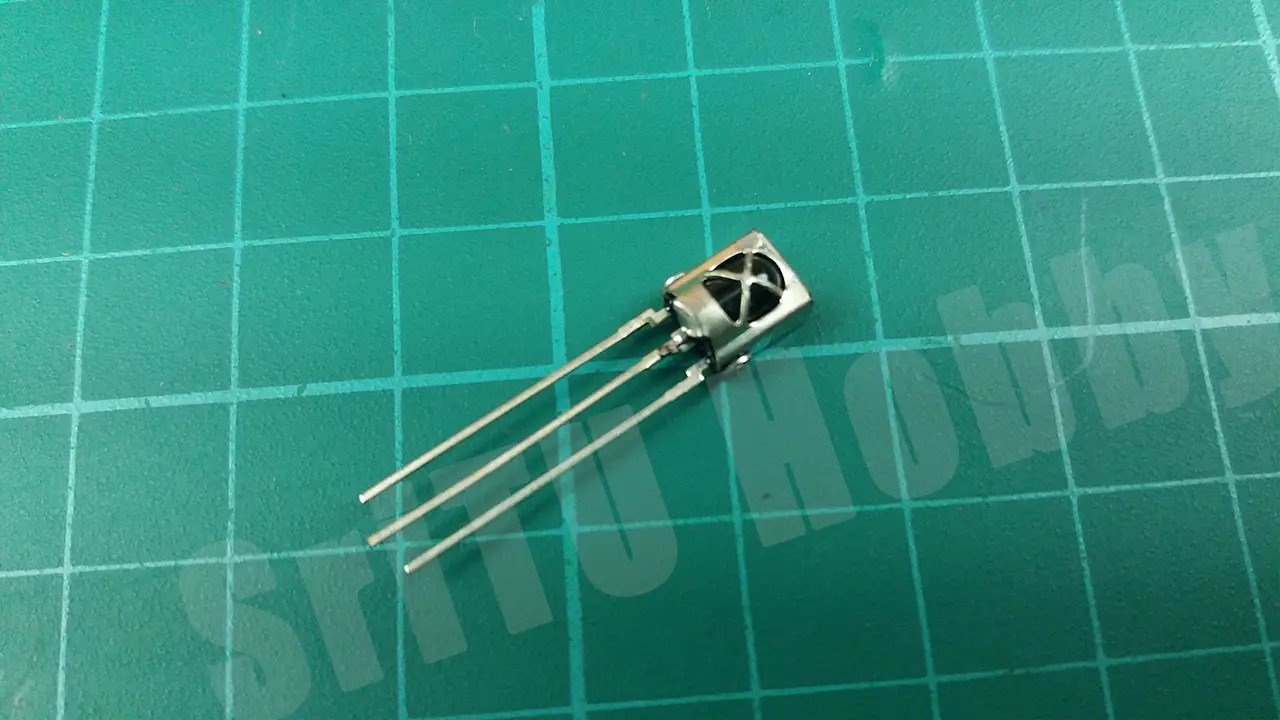LED Bulb.180-ohm Resistor.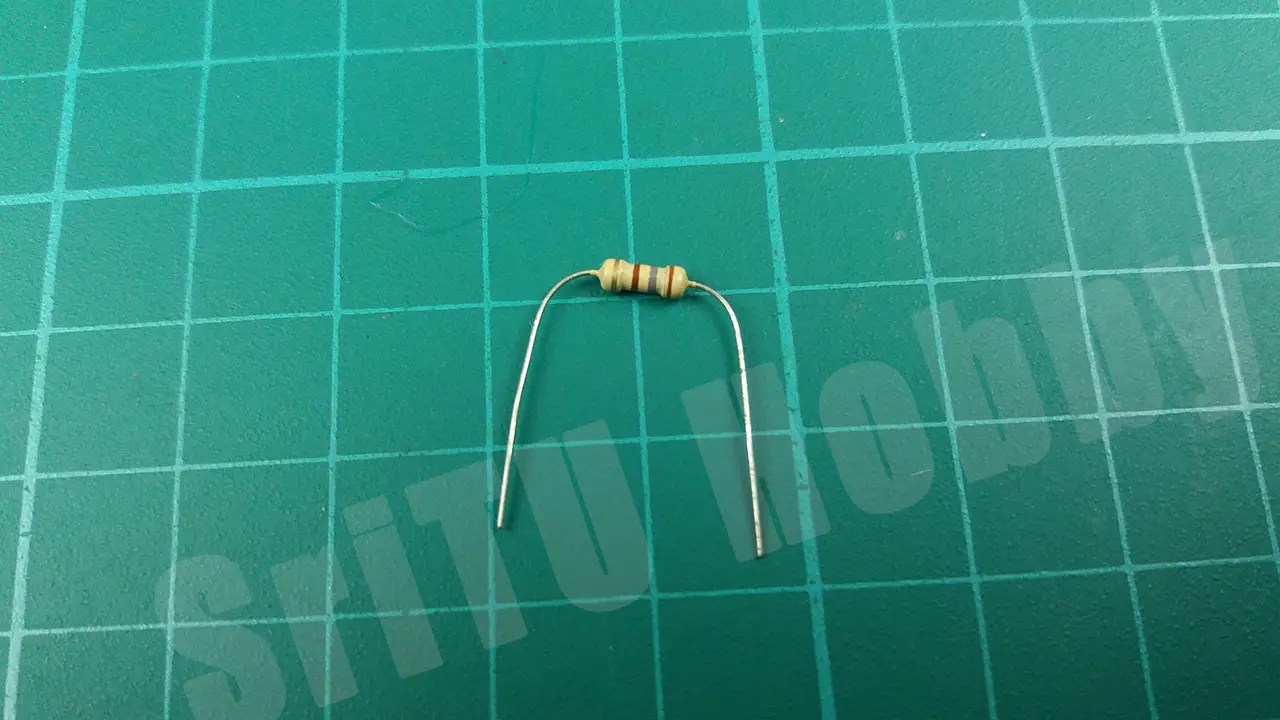5v buzzer.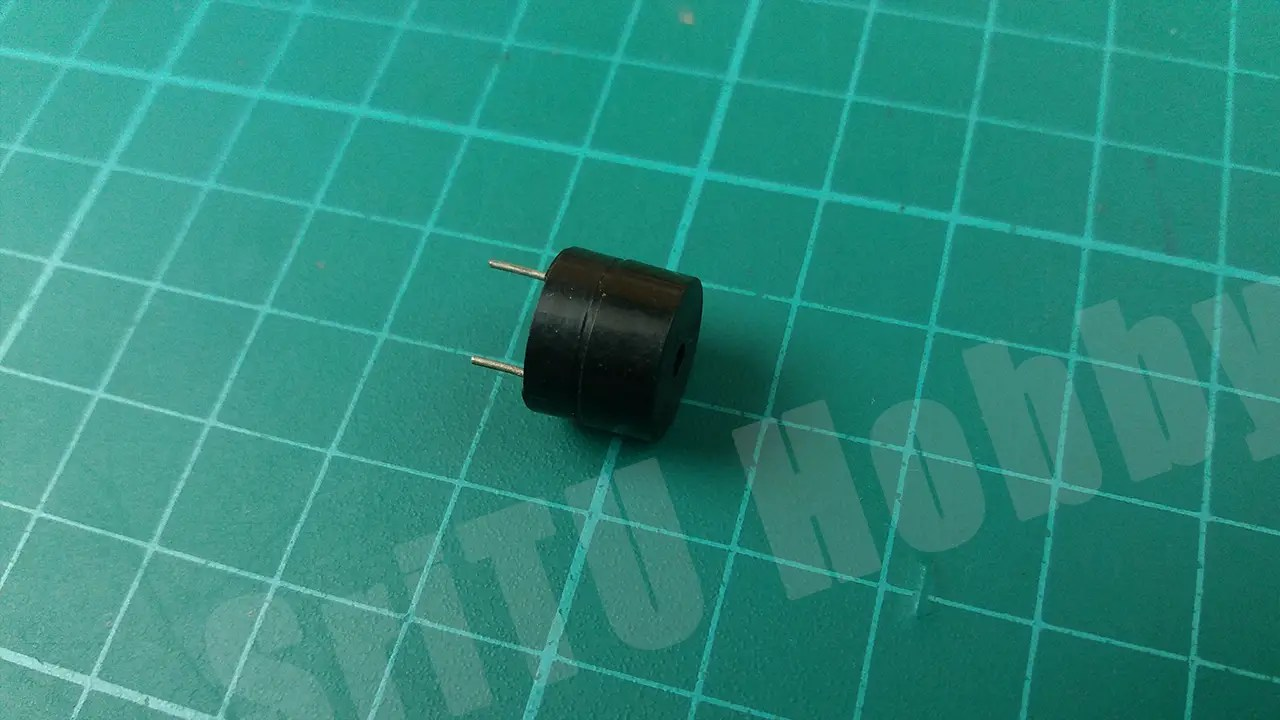Jumper wires.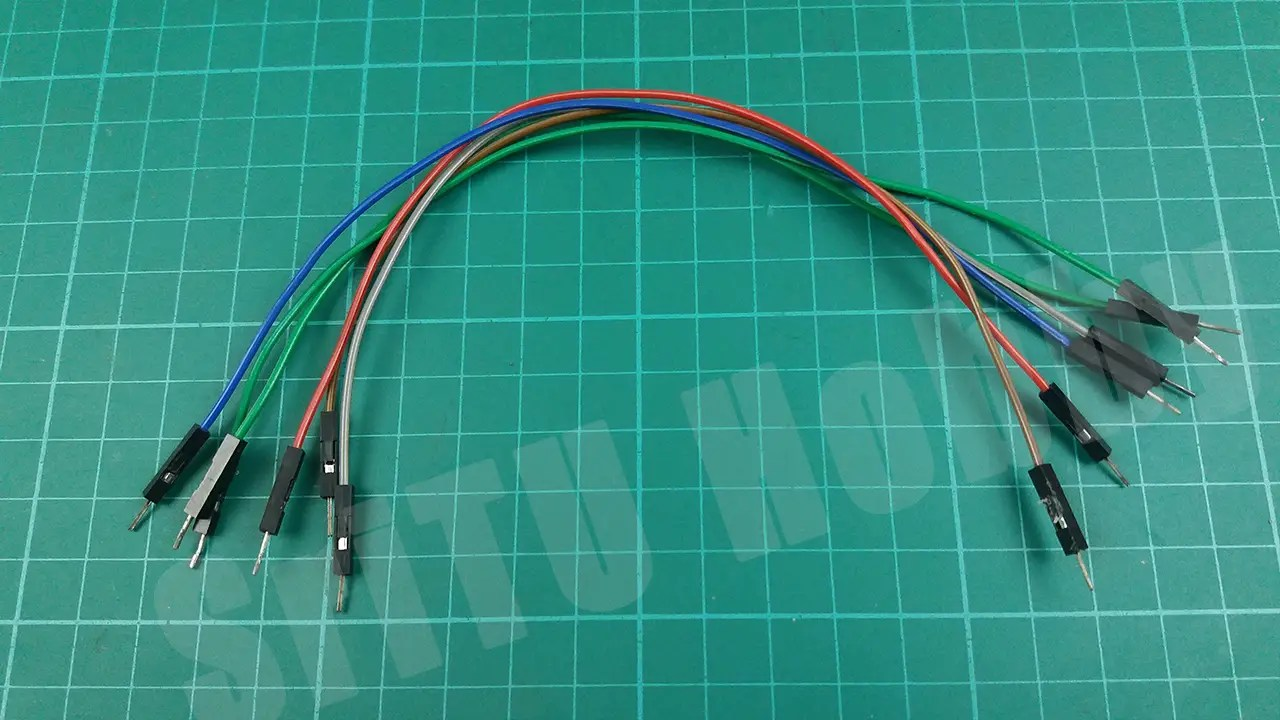IR Remote.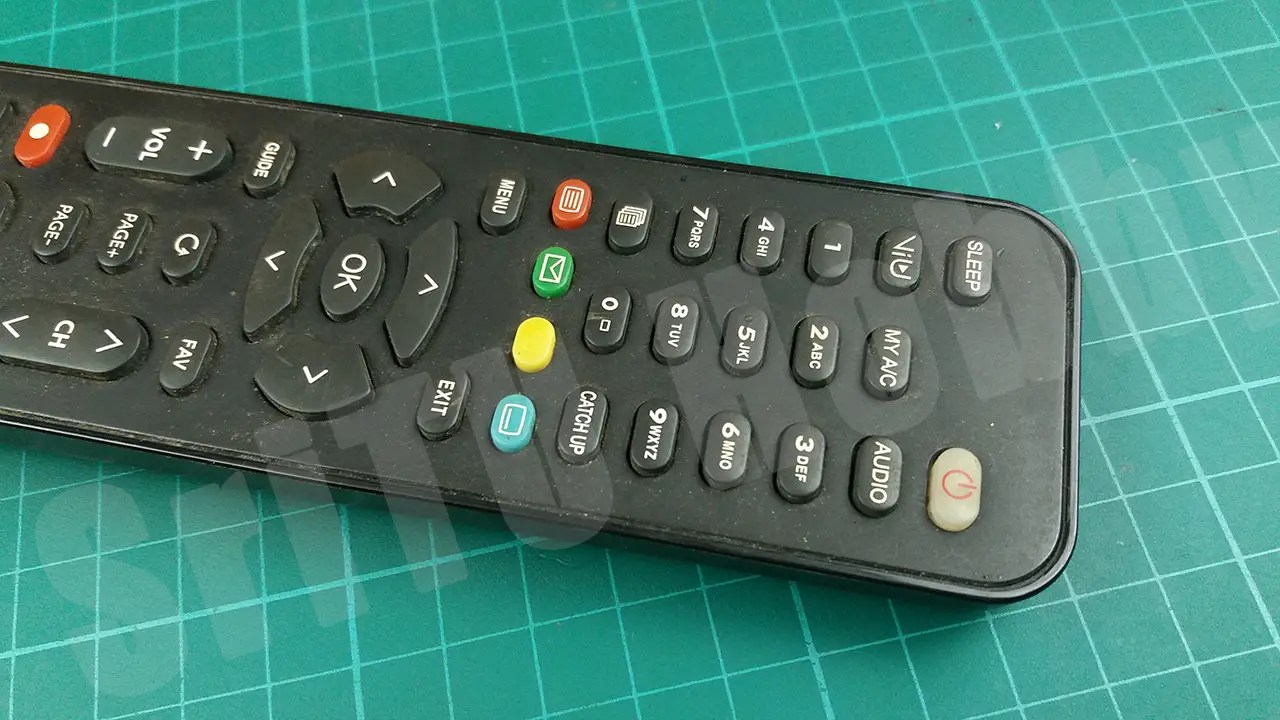### Step 2

Next, connect these components using the circuit diagram below.### Step 3

Well, now we will create the required program for this. For that, first, include the library related to the IR receiver into the Arduino IDE. Download it from the link.

### Step 4

Now we will get the values received through the IR Remote into the Arduino IDE. For that, we can use the following code.
``````/*IR receiver circuit.
created by the SriTu Tech team.
Read the code below and use it for any of your creation
*/

#include <IRremote.h>//Include library
IRrecv IR(3);//create object and define arduino pin
decode_results result;//remote values

void setup() {
Serial.begin(9600);//enable serial monitor
IR.enableIRIn();//enable IR reciver
pinMode(4, OUTPUT);//define buzzer pin
pinMode(5, OUTPUT);//define led pin

}

void loop() {
if (IR.decode(&result)) {
IR.resume();
}
delay(200);

long data = result.value;
switch (data) {
case 1: digitalWrite(4, HIGH);//press 1,buzzer on
break;
case 2: digitalWrite(4, LOW);//press 2,buzzer off
break;
case 3: digitalWrite(5, HIGH);//press 4,led on
break;
case 4: digitalWrite(5, LOW);//press 5,led off
break;
}
}``````

#### Code explanation

Here first includes the IR library and create an object for it and enter the PIN connected to the IR receiver. Then a variable called “result” is created to put the remote values.
#include <IRremote.h>//Include library
IRrecv IR(3);//create object and define arduino pin
decode_results result;//remote values
Later, the IR receiver and serial monitor are enabled in the void setup. Next LED and buzzer-connected PINs are set as OUTPUT PINs.
void setup() {
Serial.begin(9600);//enable serial monitor
IR.enableIRIn();//enable IR reciver
pinMode(4, OUTPUT);//define buzzer pin
pinMode(5, OUTPUT);//define led pin

}

Next, the values received through the Ir remote in the Void loop are retrieved and displayed on the Serial monitor.
if (IR.decode(&result)) {
IR.resume();
}
delay(200);
Later, a switch case statement was made. For that, you need to enter the IR remote values. We will do that later.
long data = result.value;
switch (data) {
case 1: digitalWrite(4, HIGH);//press 1,buzzer on
break;
case 2: digitalWrite(4, LOW);//press 2,buzzer off
break;
case 3: digitalWrite(5, HIGH);//press 4,led on
break;
case 4: digitalWrite(5, LOW);//press 5,led off
break;
}

### Step 5

Now select the correct board and port and upload it into the Arduino board. After uploading the code, RUN the serial monitor.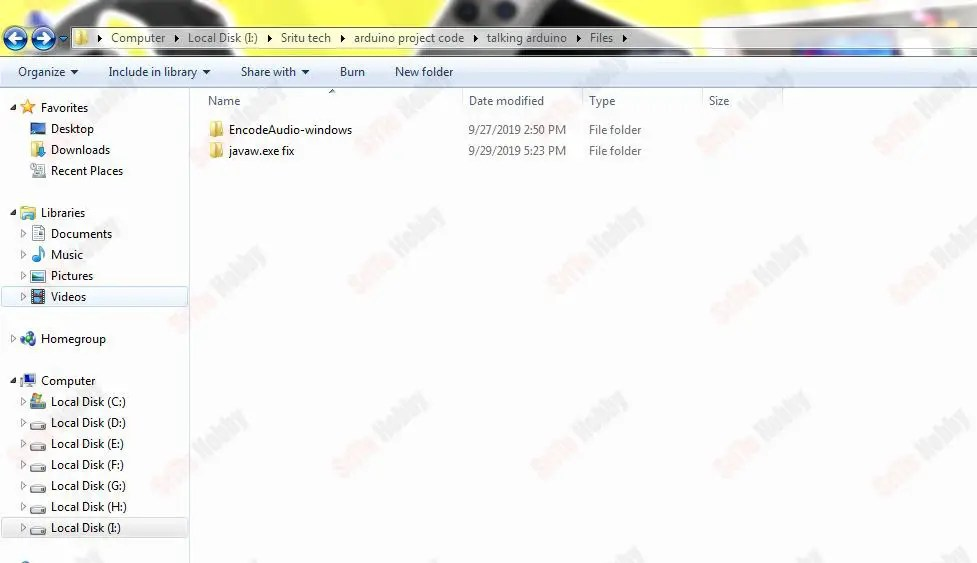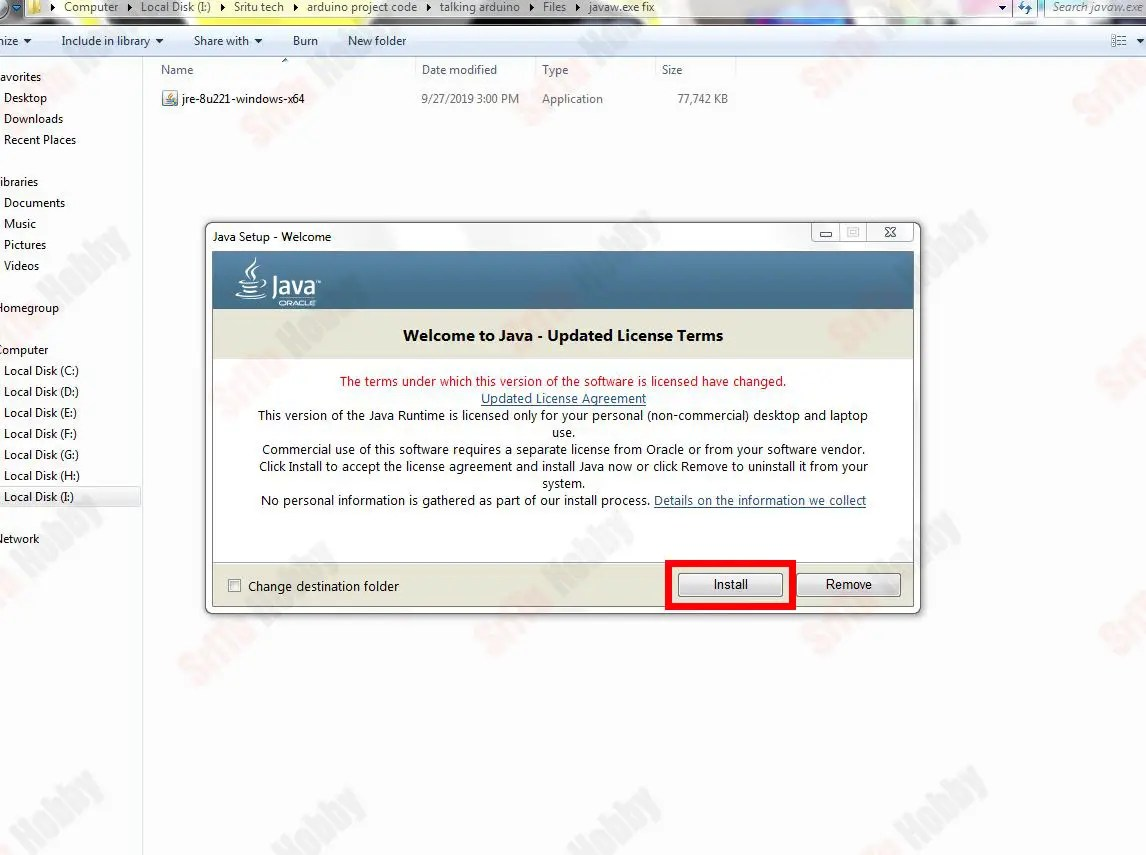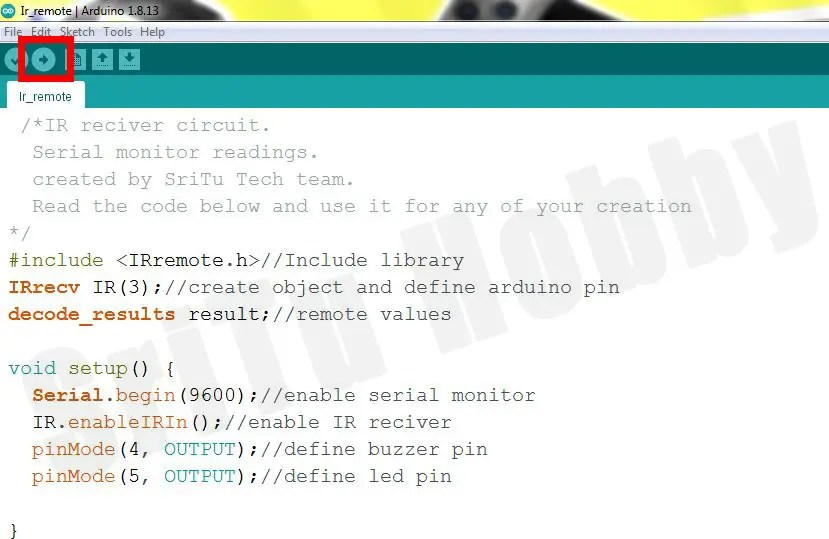### Step 6

Now let us get the values related to the buttons on the IR remote that we want to use for our project. For that, place the remote you want to use next to the IR receiver and press the desired button. Then you can see the value related to that button in the serial monitor. I have used four buttons to turn the LED ON / ON and the Buzzer on / off. You can use any four-button you like for this.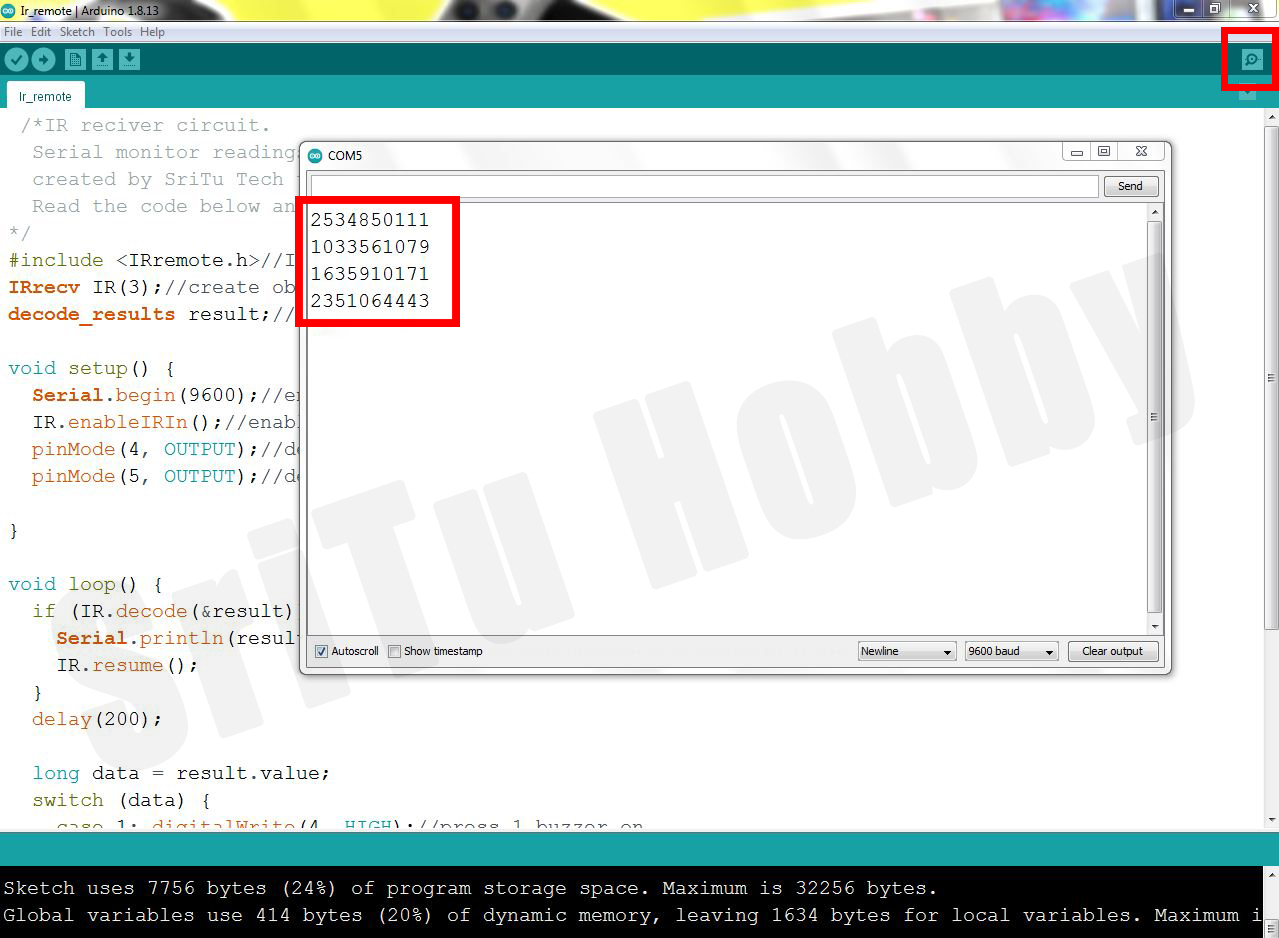### Step 7

Now let us add these values to the Switch case statement. Enter the four values as shown below.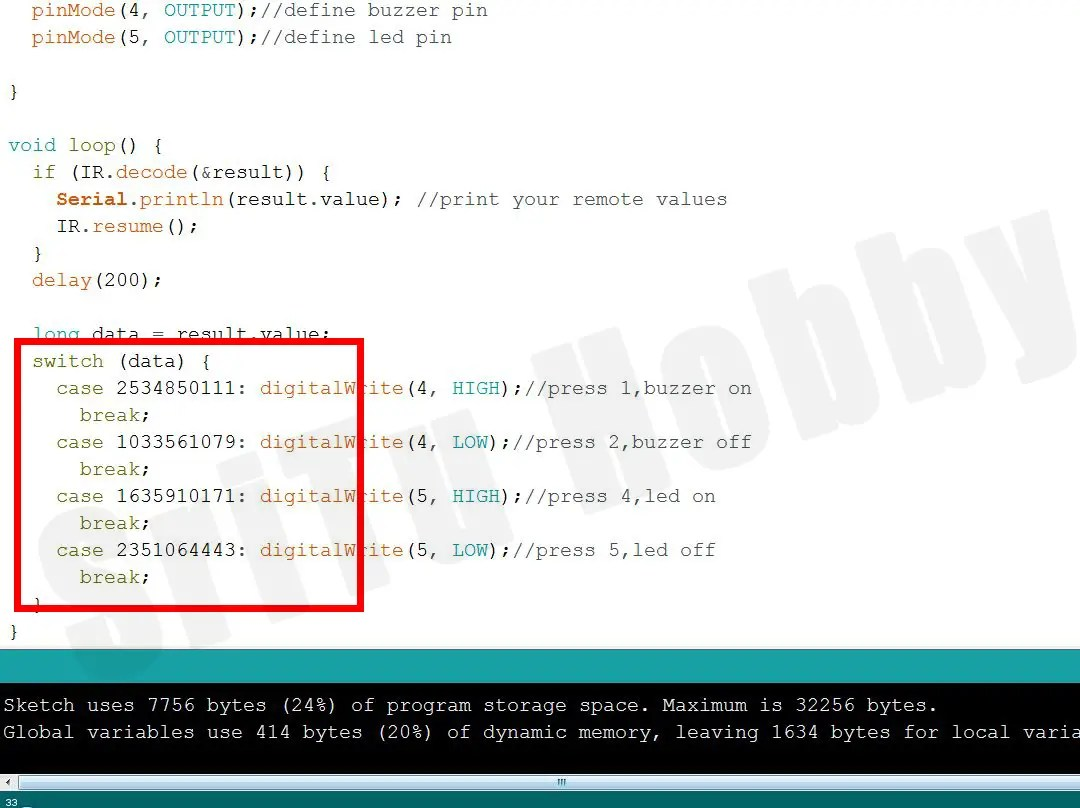### Step 8

Well, upload this code again to the Arduino board. Now we can test this project. Try the remote button you used for that. If you did this project according to the instructions above, the project will work properly. Below is the full video guide.

Well, now we will learn how to turn on / off four LED bulbs using an IR module. You can also use a relay module to replace this LED. Then you can control the electrical appliances in the house. This IR module includes an IR receiver and an IR remote. This also works in the same way as above. The accessories required for this are given below.

OK, Let’s do this project step by step.

### Step 1

Identify these components.
Arduino UNO board.IR Module.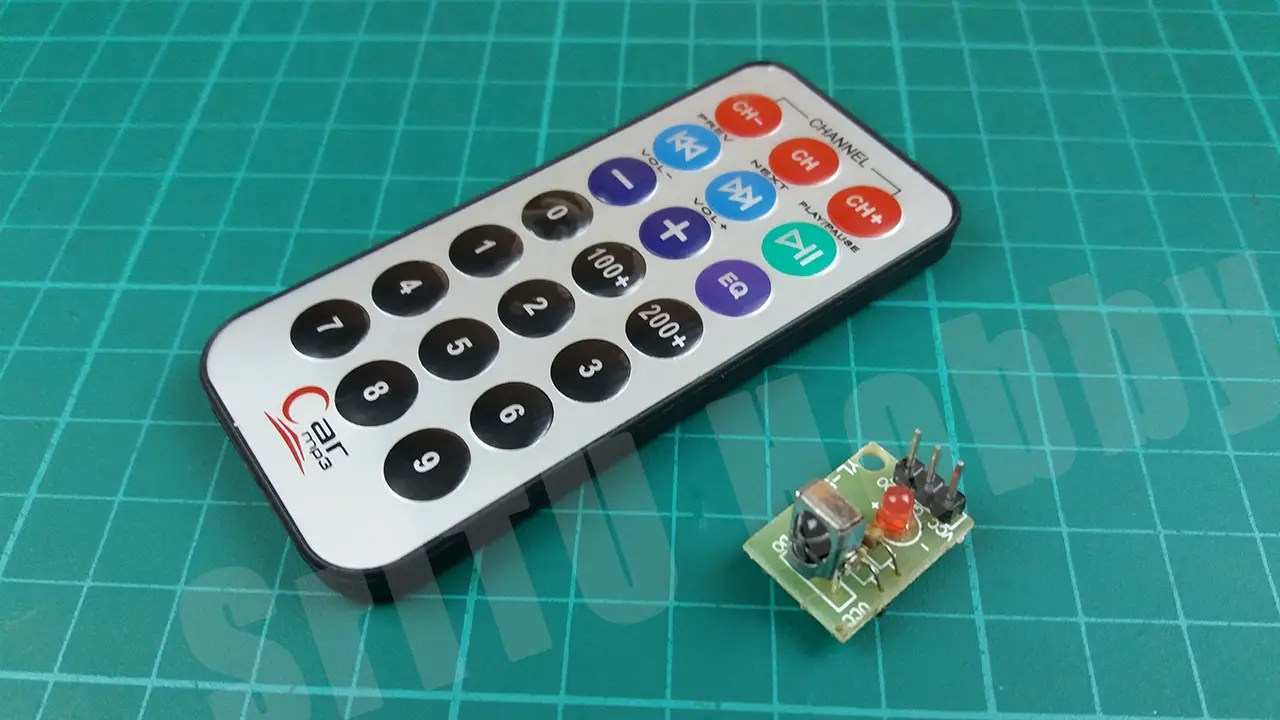LED Bulb.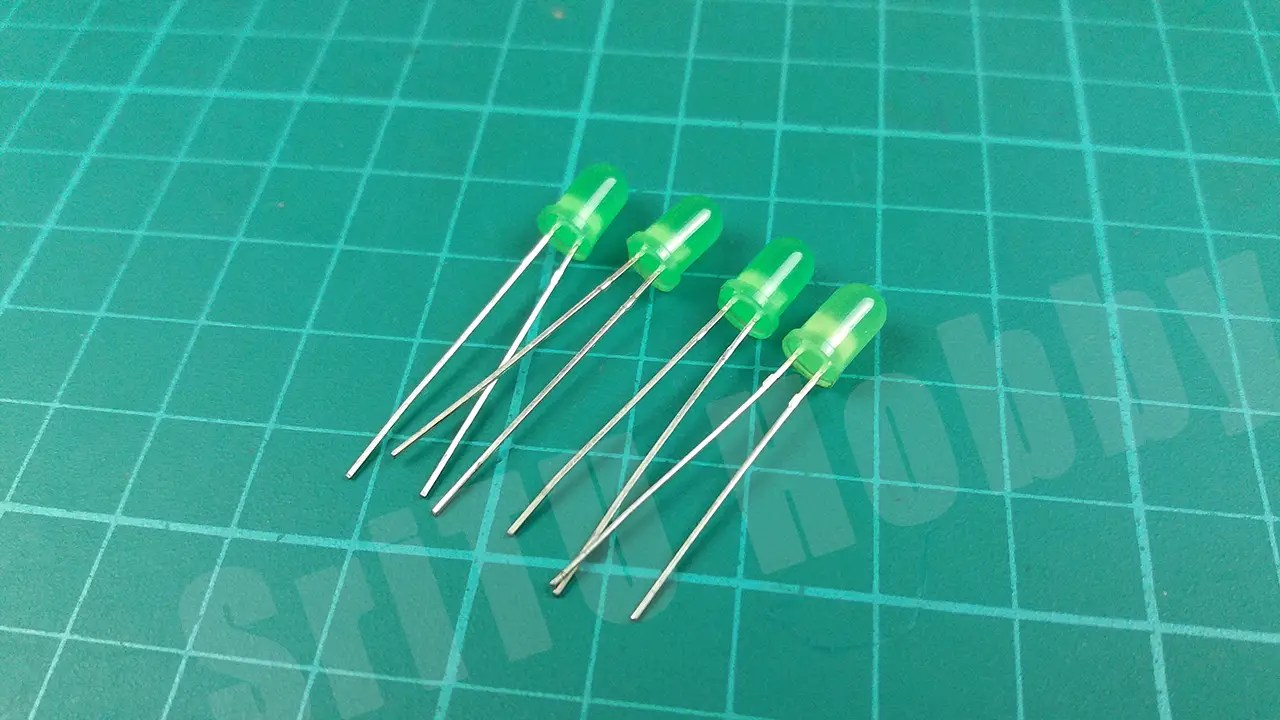180-ohm Resistor.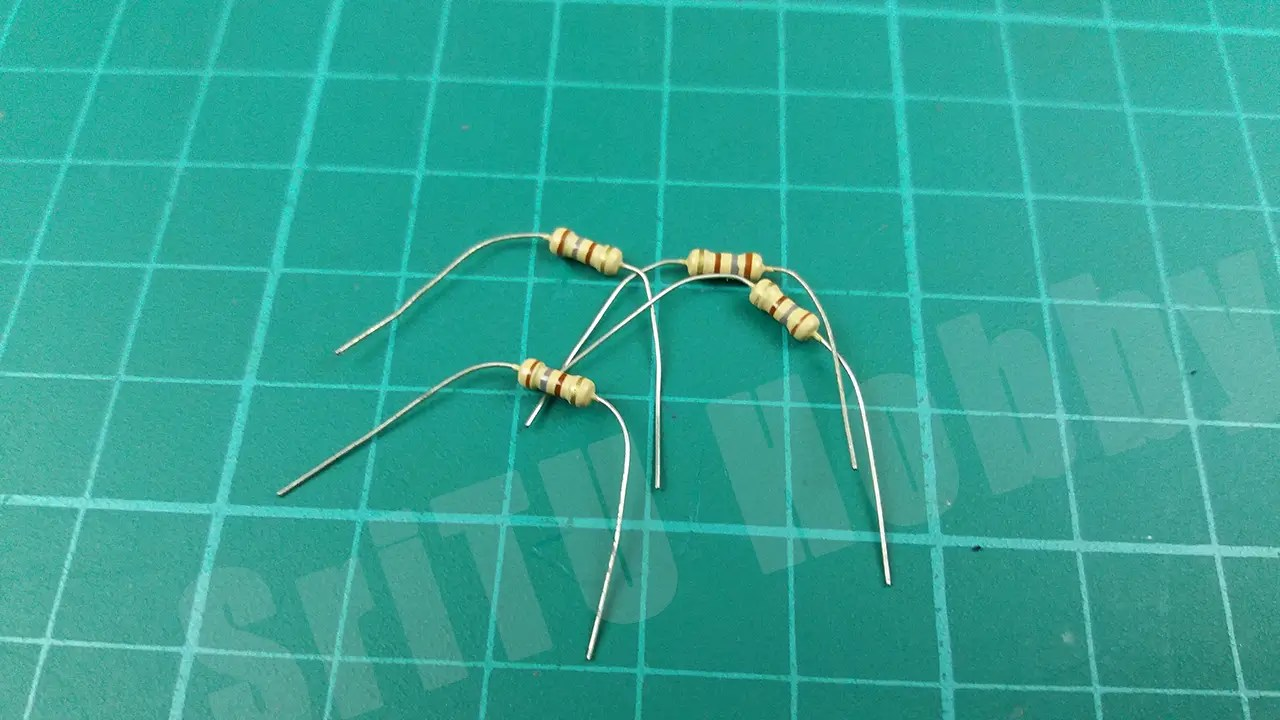Jumper wires.### Step 2

Connect these components using the circuit diagram below.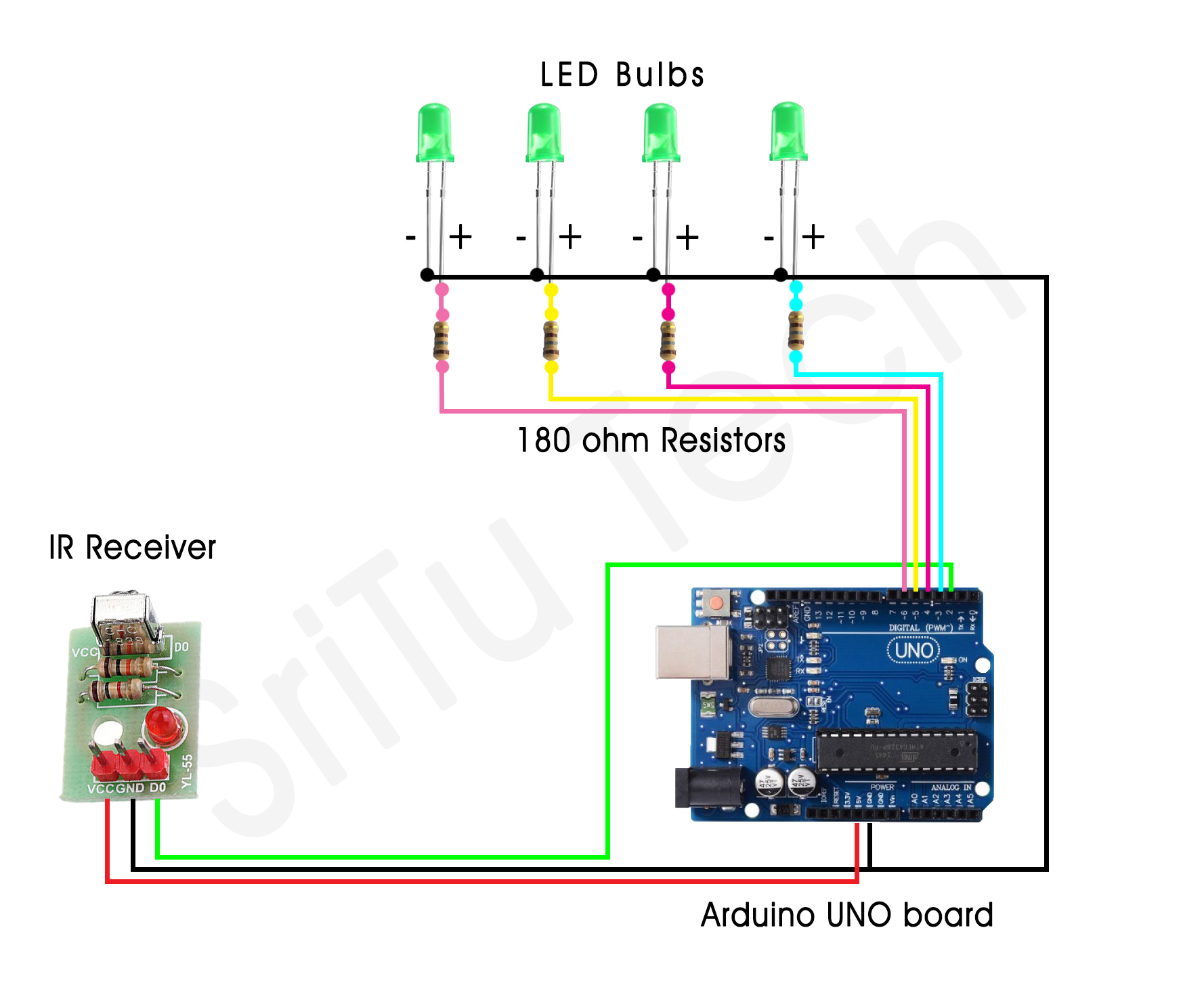### Step 3

Well, now we will create the required program for this. It is presented below.
``````/*IR receiver circuit.
created by the SriTu Tech team.
Read the code below and use it for any of your creations.
*/
#include <IRremote.h>//Include library
IRrecv IR(2);//create object and define arduino pin
decode_results result;//remote values
#define value1 0
#define value2 0
#define value3 0
#define value4 0
bool LED1 = 0;
bool LED2 = 0;
bool LED3 = 0;
bool LED4 = 0;
void setup() {
Serial.begin(9600);//enable serial monitor
IR.enableIRIn();//enable IR reciver
pinMode(3, OUTPUT);//define LED 1
pinMode(4, OUTPUT);//define LED 2
pinMode(5, OUTPUT);//define LED 3
pinMode(6, OUTPUT);//define LED 4
}
void loop() {
if (IR.decode(&result)) {
IR.resume();
}
delay(200);
if (result.value == value1 && LED1 == 0) {
digitalWrite(3, HIGH);
LED1 = 1;
result.value = 0;
} else if (result.value == value1 && LED1 == 1) {
digitalWrite(3, LOW);
LED1 = 0;
result.value = 0;
}
if (result.value == value2 && LED2 == 0) {
digitalWrite(4, HIGH);
LED2 = 1;
result.value = 0;
} else if (result.value == value2 && LED2 == 1) {
digitalWrite(4, LOW);
LED2 = 0;
result.value = 0;
}
if (result.value == value3 && LED3 == 0) {
digitalWrite(5, HIGH);
LED3 = 1;
result.value = 0;
} else if (result.value == value3 && LED3 == 1) {
digitalWrite(5, LOW);
LED3 = 0;
result.value = 0;
}
if (result.value == value4 && LED4 == 0) {
digitalWrite(6, HIGH);
LED4 = 1;
result.value = 0;
} else if (result.value == value4 && LED4 == 1) {
digitalWrite(6, LOW);
LED4 = 0;
result.value = 0;
}
}``````

#### Code explanation

In this code too, first include the IR library, create an object for it and enter the PIN number attached to the IR recipient. Then a variable called “result” is created to put the IR remote values.
#include <IRremote.h>//Include library
IRrecv IR(2);//create object and define arduino pin
decode_results result;//remote values
Then four variables are defined to put IR remote values. These should include values received via the IR remote.
#define value1 0
#define value2 0
#define value3 0
#define value4 0
Next, four bool-type variables are created to support the code.
bool LED1 = 0;
bool LED2 = 0;
bool LED3 = 0;
bool LED4 = 0;
Later, the Serial monitor and IR receiver are enabled in the Void setup. Also, the Arduino PINS connected to the LED bulb has been turned into an output pin.
void setup() {
Serial.begin(9600);//enable serial monitor
IR.enableIRIn();//enable IR reciver
pinMode(3, OUTPUT);//define LED 1
pinMode(4, OUTPUT);//define LED 2
pinMode(5, OUTPUT);//define LED 3
pinMode(6, OUTPUT);//define LED 4
}
The code for get the IR remote values in the void loop and displaying them on the serial monitor is included.
if (IR.decode(&result)) {
IR.resume();
}
delay(200);
It also checks the IR remote values by the IF condition and includes the code for turning the LEDs ON / ON.
if (result.value == value1 && LED1 == 0) {
digitalWrite(3, HIGH);
LED1 = 1;
result.value = 0;
} else if (result.value == value1 && LED1 == 1) {
digitalWrite(3, LOW);
LED1 = 0;
result.value = 0;
}
if (result.value == value2 && LED2 == 0) {
digitalWrite(4, HIGH);
LED2 = 1;
result.value = 0;
} else if (result.value == value2 && LED2 == 1) {
digitalWrite(4, LOW);
LED2 = 0;
result.value = 0;
}
if (result.value == value3 && LED3 == 0) {
digitalWrite(5, HIGH);
LED3 = 1;
result.value = 0;
} else if (result.value == value3 && LED3 == 1) {
digitalWrite(5, LOW);
LED3 = 0;
result.value = 0;
}
if (result.value == value4 && LED4 == 0) {
digitalWrite(6, HIGH);
LED4 = 1;
result.value = 0;
} else if (result.value == value4 && LED4 == 1) {
digitalWrite(6, LOW);
LED4 = 0;
result.value = 0;
}

### Step 4

Well, now we will upload this code to the Arduino board. After uploading the code, run the Serial monitor.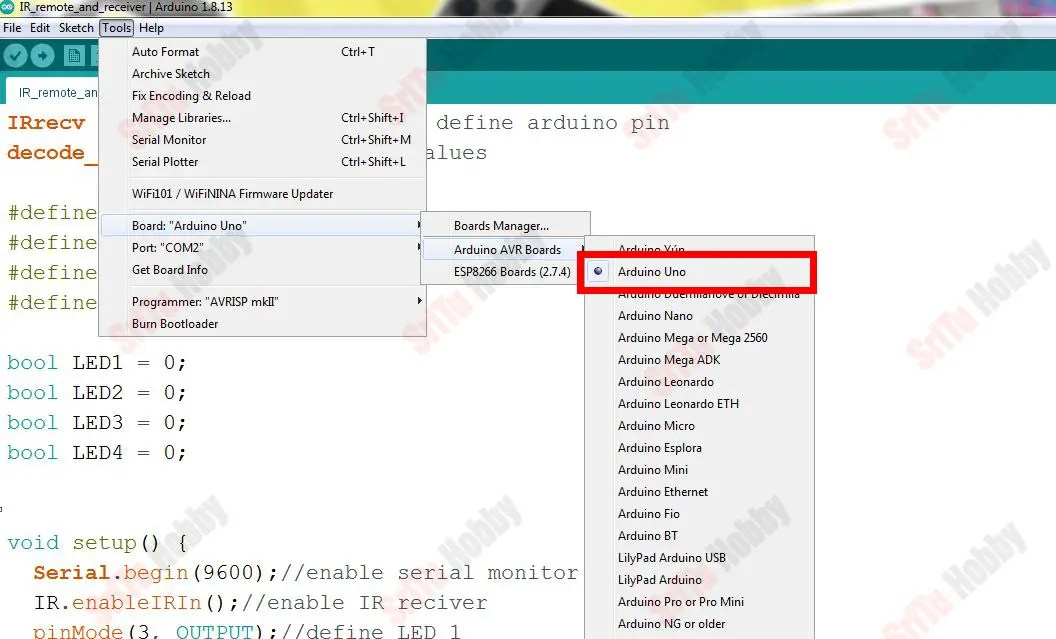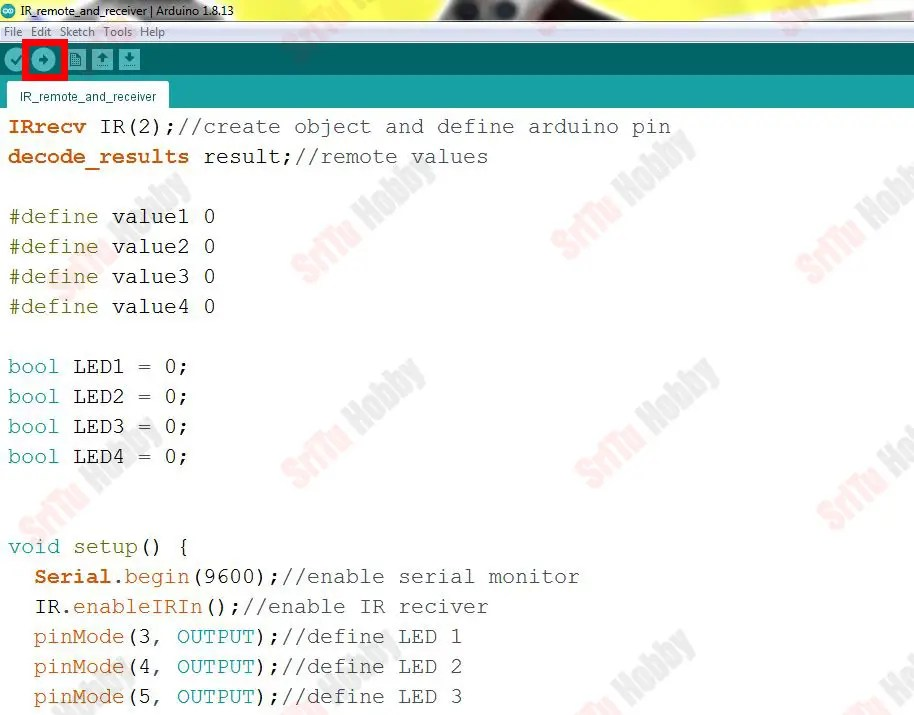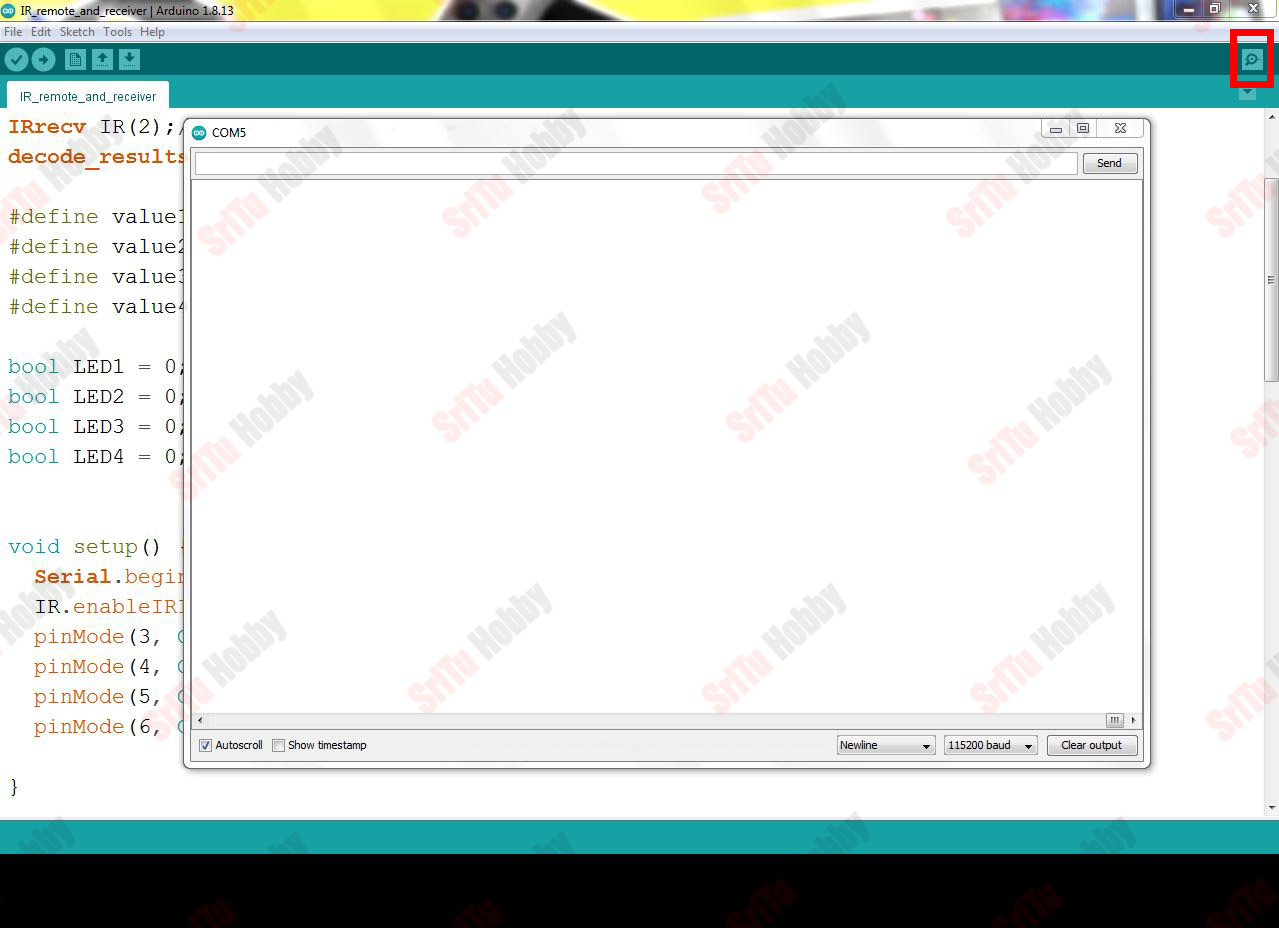### Step 5

Then place the IR remote next to the IR receiver and select the four buttons you want to turn on / off the four LEDs and press them.### Step 6

Then enter those values as follows.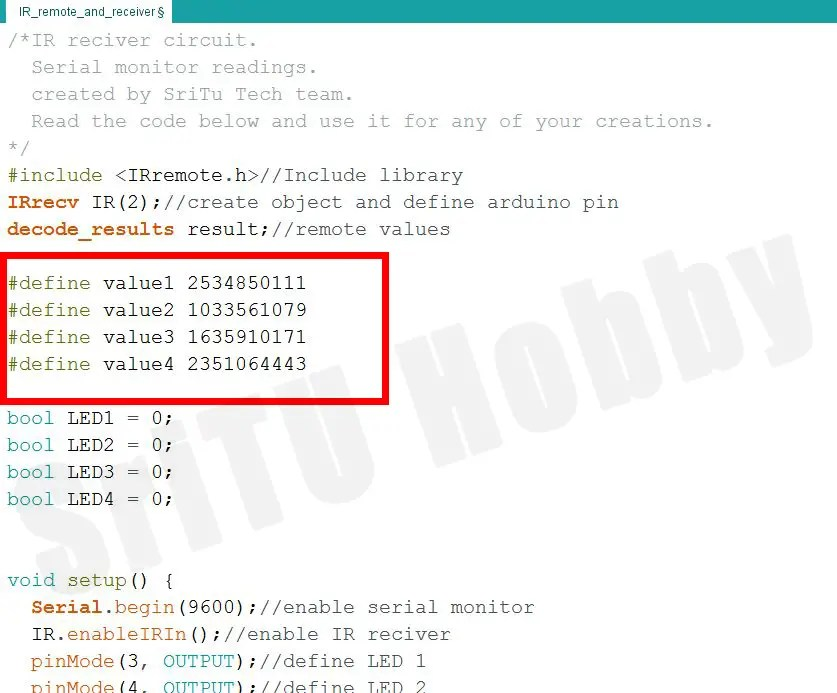### Step 7

Finally, upload this code again to the Arduino board. Now we can turn ON / OFF the four LED bulbs one by one with our IR remote. The full video guide can be viewed below.

OK, now let’s learn how to control an 8-channel relay module using an IR receiver and an IR remote. This can be done in the same way as above. The required accessories are listed below.
So, let’s do this project step by step.

### Step 1

Firstly, identify these components.

#### Arduino UNO board#### 8-channel Relay module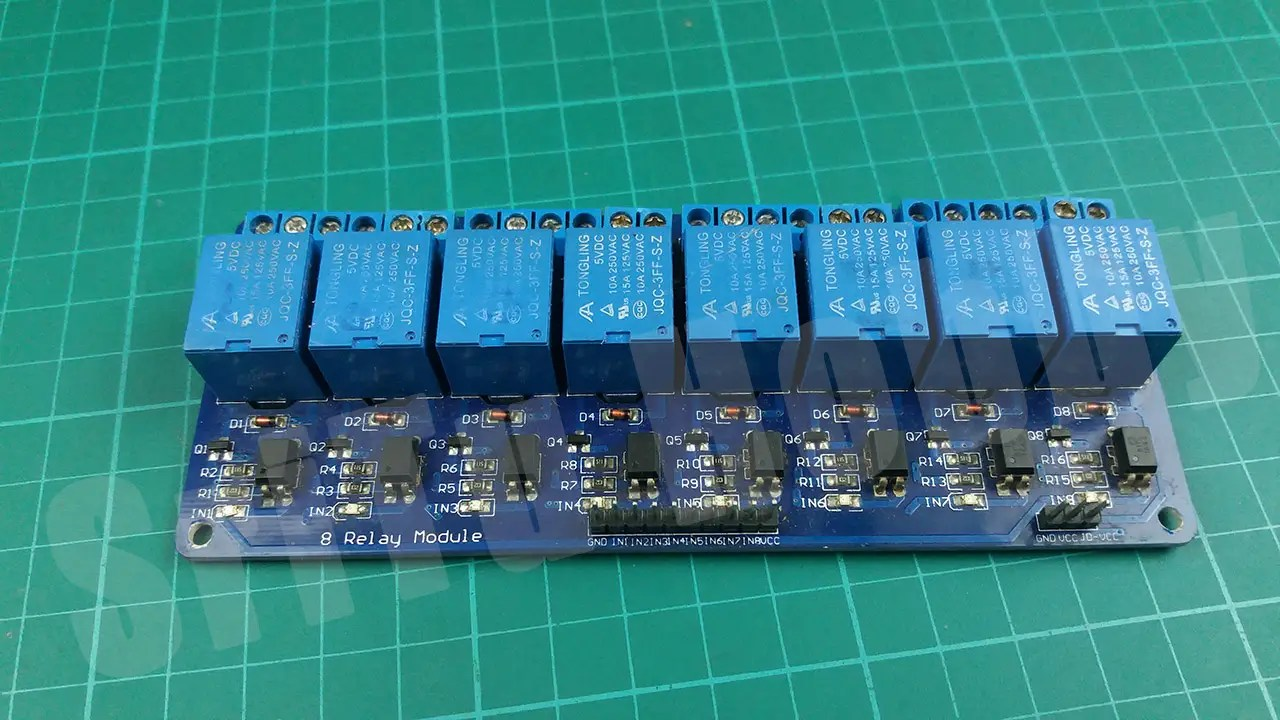#### Jumper wires### Step 2

Secondly, connect these components using the circuit diagram below. Also, remember to connect a separate external 5V power supply to the relay module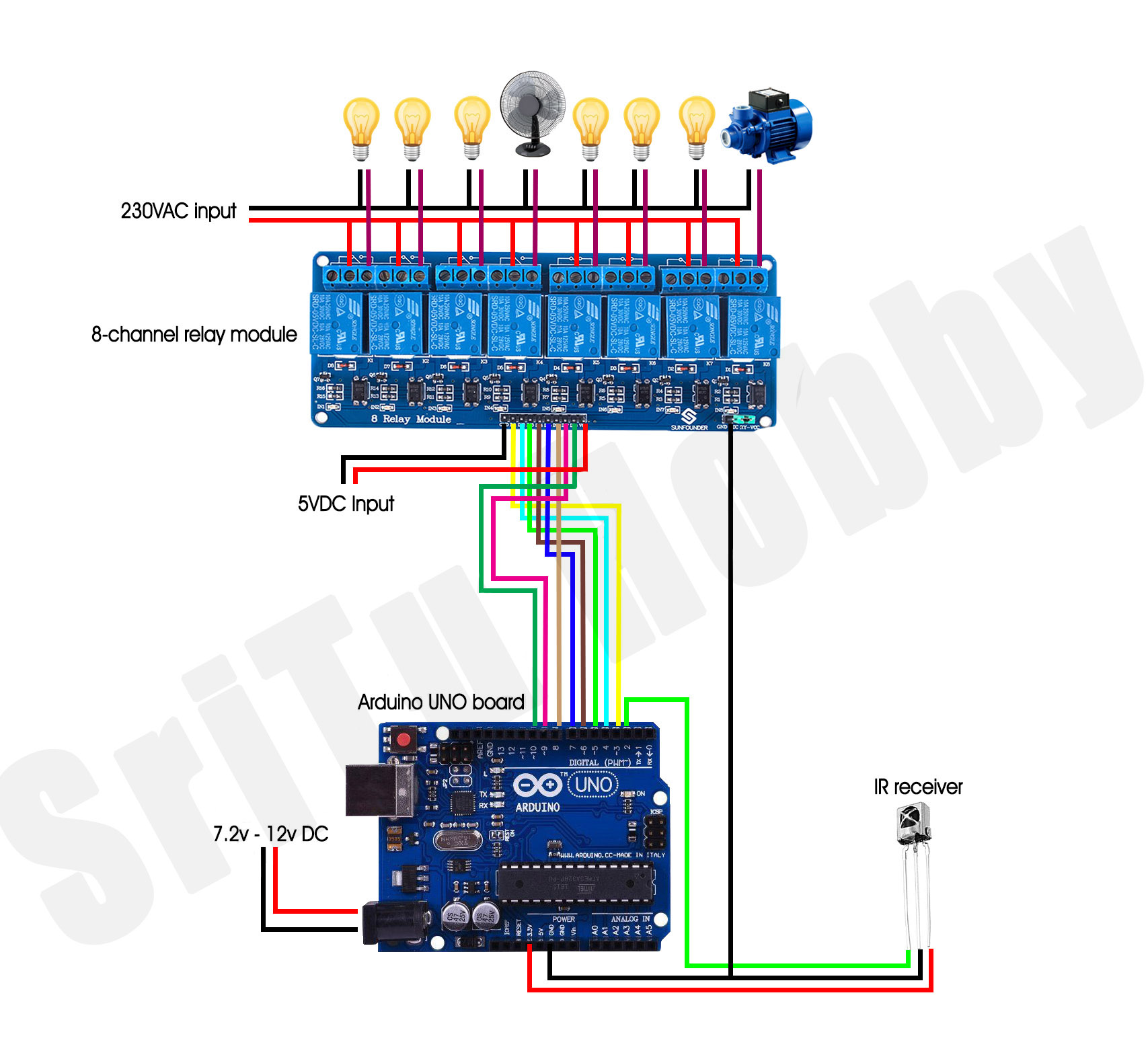### Step 3

Well now let’s make the necessary program for this. It is as follows.

``````#include <IRremote.h>
#define PIN 2
#define Relay1 3
#define Relay2 4
#define Relay3 5
#define Relay4 6
#define Relay5 7
#define Relay6 8
#define Relay7 9
#define Relay8 10
IRrecv irrecv(PIN);
decode_results results;

#define remotevalue1 ""
#define remotevalue2 ""
#define remotevalue3 ""
#define remotevalue4 ""
#define remotevalue5 ""
#define remotevalue6 ""
#define remotevalue7 ""
#define remotevalue8 ""

byte relay1 = 0;
byte relay2 = 0;
byte relay3 = 0;
byte relay4 = 0;
byte relay5 = 0;
byte relay6 = 0;
byte relay7 = 0;
byte relay8 = 0;
long value;

void setup() {
Serial.begin(9600);
irrecv.enableIRIn();
pinMode(Relay1, OUTPUT);
pinMode(Relay2, OUTPUT);
pinMode(Relay3, OUTPUT);
pinMode(Relay4, OUTPUT);
pinMode(Relay5, OUTPUT);
pinMode(Relay6, OUTPUT);
pinMode(Relay7, OUTPUT);
pinMode(Relay8, OUTPUT);

for (int a = 3; a <= 10; a++ ) {
digitalWrite(a, HIGH);
}

}

void loop() {
if (irrecv.decode(&results)) {
Serial.println(results.value);
value = (results.value);
irrecv.resume();
}
delay(400);

if (value == remotevalue1  && relay1 == 0 ) {
digitalWrite(Relay1, LOW);
relay1 = 1;
value = 0;
} else if (value == remotevalue1 && relay1 == 1 ) {
digitalWrite(Relay1, HIGH);
relay1 = 0;
value = 0;
}

if (value == remotevalue2 && relay2 == 0 ) {
digitalWrite(Relay2, LOW);
relay2 = 1;
value = 0;
} else if (value == remotevalue2 && relay2 == 1 ) {
digitalWrite(Relay2, HIGH);
relay2 = 0;
value = 0;

}

if (value == remotevalue3 && relay3 == 0 ) {
digitalWrite(Relay3, LOW);
relay3 = 1;
value = 0;
} else if (value == remotevalue3 && relay3 == 1 ) {
digitalWrite(Relay3, HIGH);
relay3 = 0;
value = 0;
}

if (value == remotevalue4 && relay4 == 0 ) {
digitalWrite(Relay4, LOW);
relay4 = 1;
value = 0;
} else if (value == remotevalue4 && relay4 == 1 ) {
digitalWrite(Relay4, HIGH);
relay4 = 0;
value = 0;
}

if (value == remotevalue5 && relay5 == 0 ) {
digitalWrite(Relay5, LOW);
relay5 = 1;
value = 0;
} else if (value == remotevalue5 && relay5 == 1 ) {
digitalWrite(Relay5, HIGH);
relay5 = 0;
value = 0;
}

if (value == remotevalue6 && relay6 == 0 ) {
digitalWrite(Relay6, LOW);
relay6 = 1;
value = 0;
} else if (value == remotevalue6 && relay6 == 1 ) {
digitalWrite(Relay6, HIGH);
relay6 = 0;
value = 0;
}

if (value == remotevalue7 && relay7 == 0 ) {
digitalWrite(Relay7, LOW);
relay7 = 1;
value = 0;
} else if (value == remotevalue7 && relay7 == 1 ) {
digitalWrite(Relay7, HIGH);
relay7 = 0;
value = 0;
}

if (value == remotevalue8 && relay8 == 0 ) {
digitalWrite(Relay8, LOW);
relay8 = 1;
value = 0;
} else if (value == remotevalue8 && relay8 == 1 ) {
digitalWrite(Relay8, HIGH);
relay8 = 0;
value = 0;
}
}``````

#### Code explanation

Firstly, the IR library included.
#include <IRremote.h>

Secondly, the sensor PIN and relay PINs are defined.
#define PIN 2
#define Relay1 3
#define Relay2 4
#define Relay3 5
#define Relay4 6
#define Relay5 7
#define Relay6 8
#define Relay7 9
#define Relay8 10

Thirdly, the PIN associated with the IR sensor is inserted into the IR library. After, a variable called “result” is created to enter the values received through the IR remote.
IRrecv irrecv(PIN);
decode_results results;
Next, eight variables are defined to put the values received via IR Remote.
#define remotevalue1 “”
#define remotevalue2 “”
#define remotevalue3 “”
#define remotevalue4 “”
#define remotevalue5 “”
#define remotevalue6 “”
#define remotevalue7 “”
#define remotevalue8 “”

Afterward, eight Boolean type variables and one long type variable are created to support this program.
byte relay1 = 0;
byte relay2 = 0;
byte relay3 = 0;
byte relay4 = 0;
byte relay5 = 0;
byte relay6 = 0;
byte relay7 = 0;
byte relay8 = 0;
long value;
The serial monitor and IR library are started in the void setup. Also, the pins connected to the relay are converted into output and all the relays are turned off using a “for loop”.
void setup() {
Serial.begin(9600);
irrecv.enableIRIn();
pinMode(Relay1, OUTPUT);
pinMode(Relay2, OUTPUT);
pinMode(Relay3, OUTPUT);
pinMode(Relay4, OUTPUT);
pinMode(Relay5, OUTPUT);
pinMode(Relay6, OUTPUT);
pinMode(Relay7, OUTPUT);
pinMode(Relay8, OUTPUT);

for (int a = 3; a <= 10; a++ ) {
digitalWrite(a, HIGH);
}
}

In the void loop, the values received over the IR remote are displayed on the serial monitor. Also, these values are added to the variable we previously named “value”.

if (irrecv.decode(&results)) {
Serial.println(results.value);
value = (results.value);
irrecv.resume();
}
delay(400);

Lastly, the IR remote values are checked by the if status, and the code is designed to activate the relay one by one.
if (value == remotevalue1  && relay1 == 0 ) {
digitalWrite(Relay1, LOW);
relay1 = 1;
value = 0;
} else if (value == remotevalue1 && relay1 == 1 ) {
digitalWrite(Relay1, HIGH);
relay1 = 0;
value = 0;
}

if (value == remotevalue2 && relay2 == 0 ) {
digitalWrite(Relay2, LOW);
relay2 = 1;
value = 0;
} else if (value == remotevalue2 && relay2 == 1 ) {
digitalWrite(Relay2, HIGH);
relay2 = 0;
value = 0;

}

if (value == remotevalue3 && relay3 == 0 ) {
digitalWrite(Relay3, LOW);
relay3 = 1;
value = 0;
} else if (value == remotevalue3 && relay3 == 1 ) {
digitalWrite(Relay3, HIGH);
relay3 = 0;
value = 0;
}

if (value == remotevalue4 && relay4 == 0 ) {
digitalWrite(Relay4, LOW);
relay4 = 1;
value = 0;
} else if (value == remotevalue4 && relay4 == 1 ) {
digitalWrite(Relay4, HIGH);
relay4 = 0;
value = 0;
}

if (value == remotevalue5 && relay5 == 0 ) {
digitalWrite(Relay5, LOW);
relay5 = 1;
value = 0;
} else if (value == remotevalue5 && relay5 == 1 ) {
digitalWrite(Relay5, HIGH);
relay5 = 0;
value = 0;
}

if (value == remotevalue6 && relay6 == 0 ) {
digitalWrite(Relay6, LOW);
relay6 = 1;
value = 0;
} else if (value == remotevalue6 && relay6 == 1 ) {
digitalWrite(Relay6, HIGH);
relay6 = 0;
value = 0;
}

if (value == remotevalue7 && relay7 == 0 ) {
digitalWrite(Relay7, LOW);
relay7 = 1;
value = 0;
} else if (value == remotevalue7 && relay7 == 1 ) {
digitalWrite(Relay7, HIGH);
relay7 = 0;
value = 0;
}

if (value == remotevalue8 && relay8 == 0 ) {
digitalWrite(Relay8, LOW);
relay8 = 1;
value = 0;
} else if (value == remotevalue8 && relay8 == 1 ) {
digitalWrite(Relay8, HIGH);
relay8 = 0;
value = 0;
}

### Step 4

OK, now select the correct board and port. Afterward, upload this code to your Arduino board and run the Serial monitor.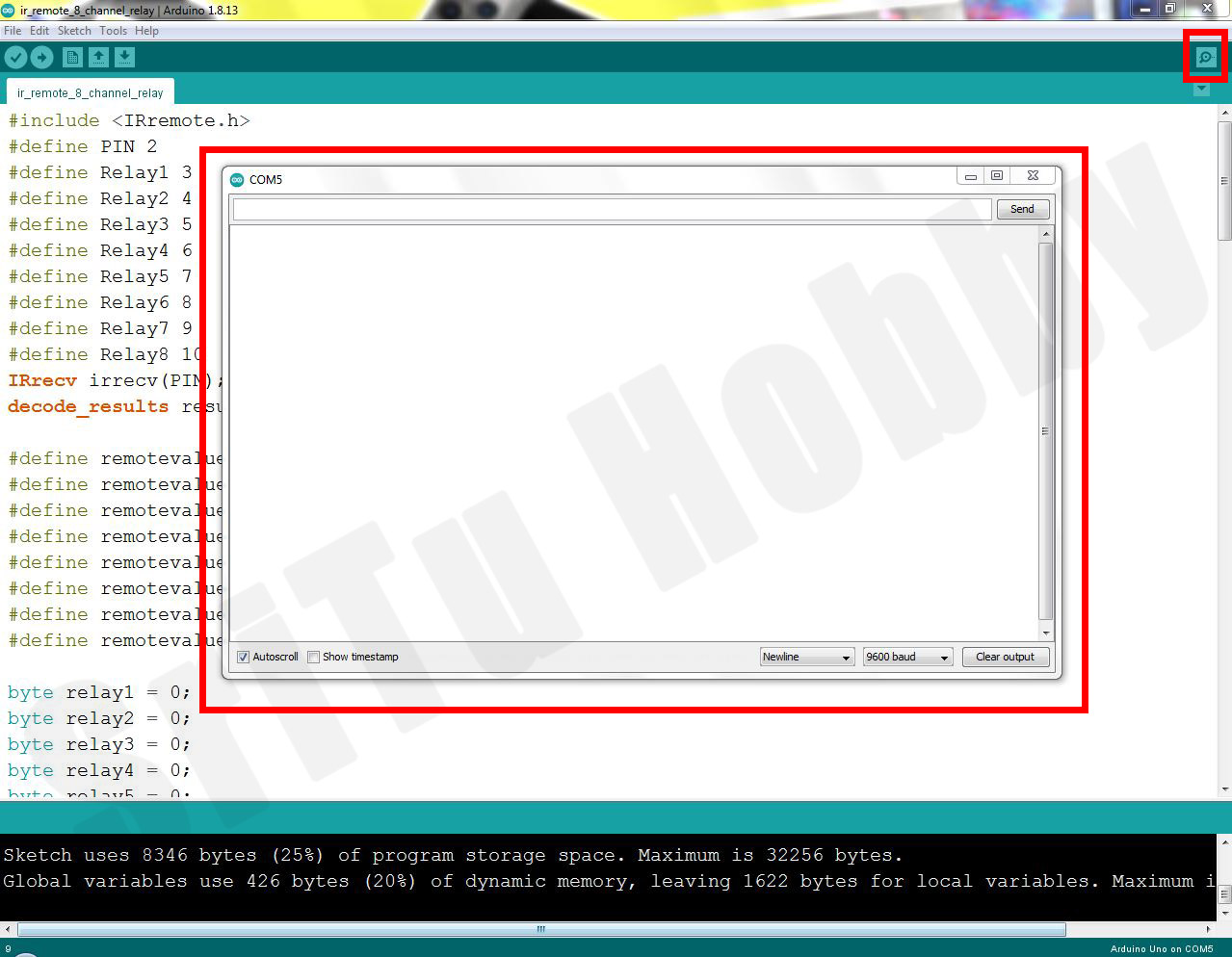### Step 5

Now, place your IR remote next to the IR receiver and press the eight buttons you like one by one. Then the IR value related to that button can be seen in the serial monitor. Now, add these values one by one to the code we defined earlier.### Step 6

Lastly, upload this code back to the Arduino board. The full video guide is given below. We will meet in the next tutorial.